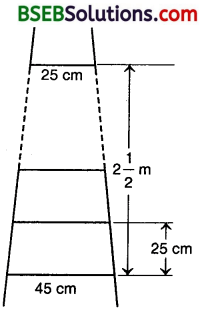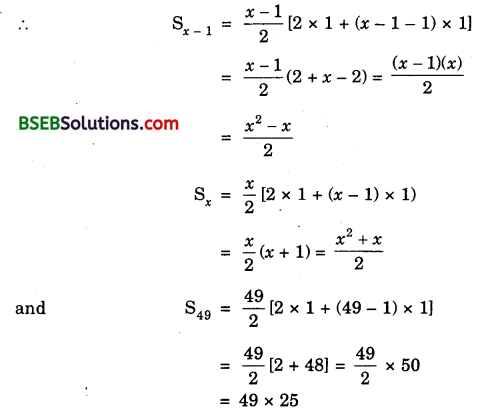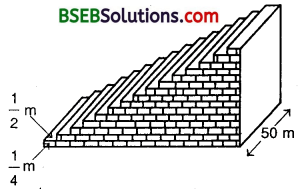# HSSlive: Plus One & Plus Two Notes & Solutions for Kerala State Board

## BSEB Class 10 Maths Chapter 5 Arithmetic Progressions Ex 5.4 Textbook Solutions PDF: Download Bihar Board STD 10th Maths Chapter 5 Arithmetic Progressions Ex 5.4 Book AnswersBSEB Class 10 Maths Chapter 5 Arithmetic Progressions Ex 5.4 Textbook Solutions PDF: Download Bihar Board STD 10th Maths Chapter 5 Arithmetic Progressions Ex 5.4 Book Answers

BSEB Class 10th Maths Chapter 5 Arithmetic Progressions Ex 5.4 Textbooks Solutions and answers for students are now available in pdf format. Bihar Board Class 10th Maths Chapter 5 Arithmetic Progressions Ex 5.4 Book answers and solutions are one of the most important study materials for any student. The Bihar Board Class 10th Maths Chapter 5 Arithmetic Progressions Ex 5.4 books are published by the Bihar Board Publishers. These Bihar Board Class 10th Maths Chapter 5 Arithmetic Progressions Ex 5.4 textbooks are prepared by a group of expert faculty members. Students can download these BSEB STD 10th Maths Chapter 5 Arithmetic Progressions Ex 5.4 book solutions pdf online from this page.

## Bihar Board Class 10th Maths Chapter 5 Arithmetic Progressions Ex 5.4 Books Solutions

 Board BSEB Materials Textbook Solutions/Guide Format DOC/PDF Class 10th Subject Maths Chapter 5 Arithmetic Progressions Ex 5.4 Chapters All Provider Hsslive

## How to download Bihar Board Class 10th Maths Chapter 5 Arithmetic Progressions Ex 5.4 Textbook Solutions Answers PDF Online?

2. Click on the Bihar Board Class 10th Maths Chapter 5 Arithmetic Progressions Ex 5.4 Answers.
3. Look for your Bihar Board STD 10th Maths Chapter 5 Arithmetic Progressions Ex 5.4 Textbooks PDF.
4. Now download or read the Bihar Board Class 10th Maths Chapter 5 Arithmetic Progressions Ex 5.4 Textbook Solutions for PDF Free.

## BSEB Class 10th Maths Chapter 5 Arithmetic Progressions Ex 5.4 Textbooks Solutions with Answer PDF Download

Find below the list of all BSEB Class 10th Maths Chapter 5 Arithmetic Progressions Ex 5.4 Textbook Solutions for PDF’s for you to download and prepare for the upcoming exams:

## BSEB Bihar Board Class 10th Maths Solutions Chapter 5 Arithmetic Progressions Ex 5.4

Question 1.
Which term of the AP : 121, 117, 113, …, is its first negative term?
Solution:
121, 117, 113, …
a = 121, d = 117 – 121 = – 4
an = a + (n – 1 )d
= 121 + in – 1) x (- 4)
= 121 – 4n + 4 = 125 – 4n
For the first negative term.
an < 0 i.e., 125 – 4n < 0
or 125 < 4n or 1254 < n
or 3114 < n n is an integer and n >3114
∴ The first negative term is 32nd term.

Question 2.
The sum of the third and the seventh terms of an AP is 6 and their product is 8. Find the sum of first sixteen terms of the AP.
Solution:
Let the AP be a – 4d, a – 3d, a – 2d, a – d, a, a + d, a + 2d, a + 3d, …
Then, a3 = a – 2d, a7 = a – 2d
So, a3+a7 = a – 2d + a – 2d = 6
or 2a = 6 or a – 3
Also, (a – 2d)(a + 2d) = 8
So, a²-4d² = 8 or 4d² = a² – 8
or 4d² = (3)² – 8 = 9 – 8 = 1
or d² = 14 or d = ± 12
Taking d = 12Question 3.
A ladder has rungs 25 cm apart (see figure). The rungs decrease uniformly in length from 45 cm, at the bottom to 25 cm at the top. If the top and the
bottom rungs are 212 m apart, what is the length of the wood required for the rungs?Solution:
Number of rungs, n = 212 m25 cm = 250 cm25 cm = 10
So, there are 10 rungs.
The length of the wood required for rungs
= sum of 10 rungs
= 102 [25 + 45] cm
= 5 x 70 cm
= 350 cm.

Question 4.
The houses of a row are numbered consecutively from 1 to 49. Show that there is a value of x such that the sum of the numbers of the houses preceding the * house numbered x is equal to the sum of the numbers of the houses following it. Find this value of x.
Solution:According to the question,∵ x is a counting number, so taking positive square root, x = 7 x 5 = 35.

Question 5.
A small terrace at a football ground comprises 15 steps each of which is 50 m long and built of solid concrete. Each step has a rise of 14 m and a tread of 12 (see figure). Calculate the total volume of concrete required to build the terrace.Solution:
Volume of concrete required to build the first step, second step, third step, … (in m³) are
14 x 12 x 50, ( 2 x 14 ) x 12 x 50, (3 x 14) x 12 x 50, ….
i.e., 508 x 2 x 508, 3 x 508, ….
∴ Total volume of concrete required
= [508 + 2 x 508 + 3 x 508 + … ] m³
= 508[1 + 2 + 3 + …] m³
= 508 x 508 [2 x 1 + (15 – 1) x 1] m³ [∵ n = 15]
= 508 x 508 x 16 = 750 m³

## Bihar Board Class 10th Maths Chapter 5 Arithmetic Progressions Ex 5.4 Textbooks for Exam Preparations

Bihar Board Class 10th Maths Chapter 5 Arithmetic Progressions Ex 5.4 Textbook Solutions can be of great help in your Bihar Board Class 10th Maths Chapter 5 Arithmetic Progressions Ex 5.4 exam preparation. The BSEB STD 10th Maths Chapter 5 Arithmetic Progressions Ex 5.4 Textbooks study material, used with the English medium textbooks, can help you complete the entire Class 10th Maths Chapter 5 Arithmetic Progressions Ex 5.4 Books State Board syllabus with maximum efficiency.

## FAQs Regarding Bihar Board Class 10th Maths Chapter 5 Arithmetic Progressions Ex 5.4 Textbook Solutions

#### Can we get a Bihar Board Book PDF for all Classes?

Yes you can get Bihar Board Text Book PDF for all classes using the links provided in the above article.

## Important Terms

Bihar Board Class 10th Maths Chapter 5 Arithmetic Progressions Ex 5.4, BSEB Class 10th Maths Chapter 5 Arithmetic Progressions Ex 5.4 Textbooks, Bihar Board Class 10th Maths Chapter 5 Arithmetic Progressions Ex 5.4, Bihar Board Class 10th Maths Chapter 5 Arithmetic Progressions Ex 5.4 Textbook solutions, BSEB Class 10th Maths Chapter 5 Arithmetic Progressions Ex 5.4 Textbooks Solutions, Bihar Board STD 10th Maths Chapter 5 Arithmetic Progressions Ex 5.4, BSEB STD 10th Maths Chapter 5 Arithmetic Progressions Ex 5.4 Textbooks, Bihar Board STD 10th Maths Chapter 5 Arithmetic Progressions Ex 5.4, Bihar Board STD 10th Maths Chapter 5 Arithmetic Progressions Ex 5.4 Textbook solutions, BSEB STD 10th Maths Chapter 5 Arithmetic Progressions Ex 5.4 Textbooks Solutions,
Share: